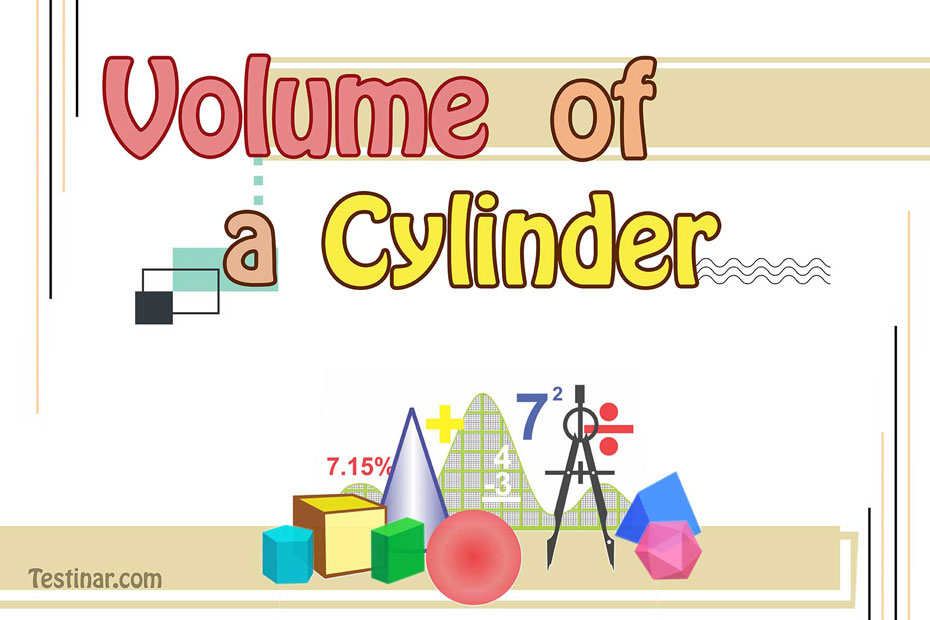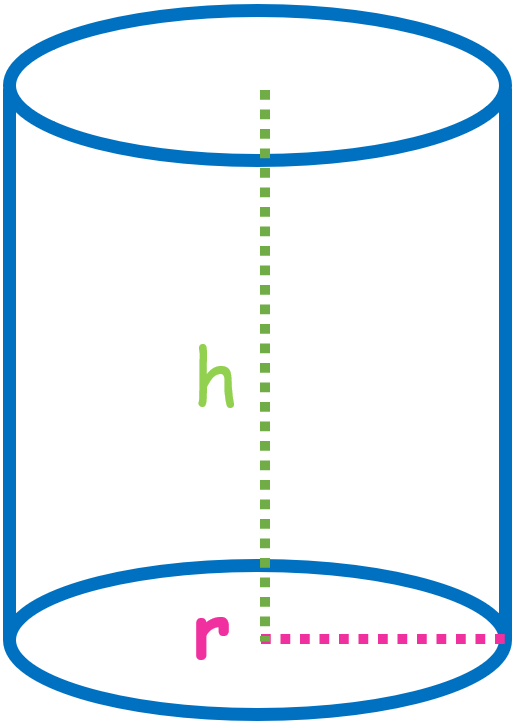## How to Find the Volume of a Cylinder

### Definition

A cylinder is a frequently seen three-dimensional solid in our environment, similar to the glue sticks we use for our craft projects, the soft drink cans we use to satisfy our thirst, or the batteries we use in our remote controls. Looking at these things we see every day, we can say that a cylinder has two circular bases, one at the top and one at the bottom. These two bases are circles that look and are the same size and shape. Line segments connect these two bases, giving the cylinder its form.### A cylinder's volume:

Volume tells us how much space is inside a cylinder. For illustration's sake, let's say we want to know how much water a cylindrical jar can store; in this case, we will need to see the jar's volume. Find the product of the base area $$(A)$$ and the cylinder height $$(h)$$. This will give you the volume $$(V)$$.

If you know the diameter and height of a cylinder, follow these steps to find its volume:

• Step 1: Look at the diagram and figure out the base's radius $$(r)$$ or diameter $$(d)$$ and the given cylinder's height $$(h)$$.
• Step 2: Divide the diameter by $$2$$ to find the radius of the base.
• Step 3: Use the given numbers in the formula to figure out the volume.
$$V \ = \ π \times r^2 \times h$$ gives the volume of the cylinder.

### Example:

Emma got a tumbler in the shape of a cylinder at Amazon. The tumbler is $$19$$ cm in diameter and $$73$$ cm tall. Find the volume of the tumbler.

Solution:

• Step1:
The tumbler is about $$19$$ cm across.
The tumbler stands $$73$$ cm tall.
• Step2:
$$r \ = \ d \ \div \ 2 \ = \ 19 \ \div \ 2 \ = \ 9.5$$
• Step 3:
How much space the cylinder takes up is: $$V \ = \ π \times r^2 \times h$$
The cylindrical tumbler's volume is: $$V \ = \ π \times (9.5)^2 \times 73$$

### Exercises for Volume of a Cylinder

1)2)3)4)5)6)7)8)9)10)1) $$\color{red}{141.3}$$ m$$^3$$2) $$\color{red}{78.5}$$ mm$$^3$$3) $$\color{red}{401.92}$$ m$$^3$$4) $$\color{red}{18.84}$$ mm$$^3$$5) $$\color{red}{452.16}$$ in$$^3$$6) $$\color{red}{100.48}$$ cm$$^3$$7) $$\color{red}{785}$$ m$$^3$$8) $$\color{red}{21.98}$$ cm$$^3$$9) $$\color{red}{226.08}$$ m$$^3$$10) $$\color{red}{150.72}$$ m$$^3$$## Volume of a Cylinder Practice Quiz

### FTCE Math Practice Workbook 2022

$25.99$12.99

### SSAT Upper Level Math for Beginners

$24.99$14.99

### Accuplacer Math for Beginners

$24.99$13.99

### 5 Full Length SSAT Lower Level Math Practice Tests

$16.99$11.99